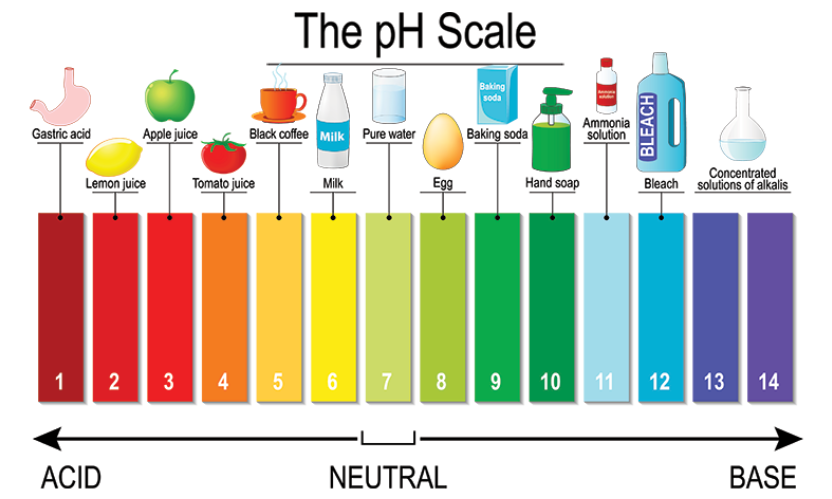# What colour will universal indicator show if you add it to the following substances?(a) Potassium hydroxide, pH = 12(b) Soda water, pH = 5(c) Sulphuric acid, pH = 2

The colour shown by a universal indicator is given below:
(a) Potassium hydroxide, pH = 12, shows dark purple colour.
(b) Soda water, pH = 5, shows orange-yellow colour.
(c) Sulphuric acid, pH = 2, shows red colour.

(Refer image for better understanding)(The pH of a solution is a measure of the molar concentration of hydrogen ions in the solution and as such is a measure of the acidity or basicity of the solution.

The numerical value for pH is just the negative of the power of 10 of the molar concentration of H+ ions.

Acidic and basic are two extremes that describe a chemical property chemical. The pH scale measures how acidic or basic a substance is.

The pH scale ranges from 0 to 14.  A pH less than 7 is acidic in nature, A pH of 7 is neutral while a pH greater than 7 is basic in nature.

pH decreases with an increase in temperature.)

Updated on: 10-Oct-2022

208 Views